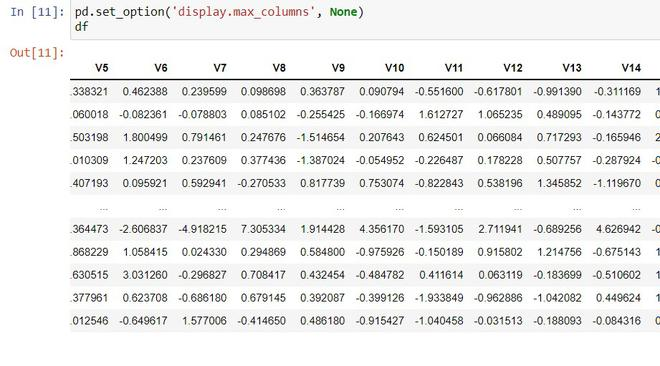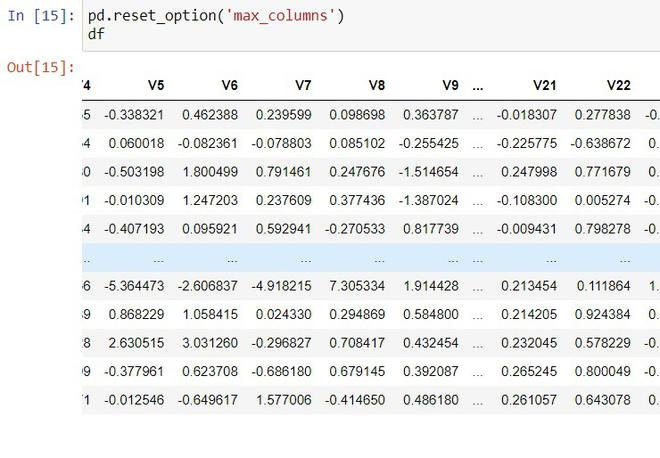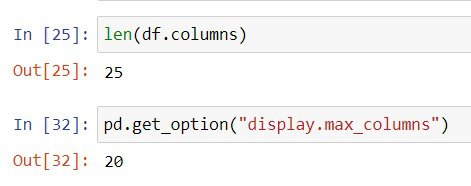Open in App
Not now

# Show all columns of Pandas DataFrame in Jupyter Notebook

• Last Updated : 30 Nov, 2021

In this article, we will discuss how to show all the columns of a pandas data frame in jupyter notebook.

Pandas have a very handy method called get option(), by this method, we can customize the output screen and work without any inconvenient form of outputs. set_option() used to set the value. This is used to set the maximum number of columns and rows that should be displayed, By setting the max_columns to None or a specified number of column

Syntax:

Syntax: pd.set_option(‘display.max_columns’, None)

## Python3

 `# importing pandas``import` `pandas as pd` `# reading csv``df ``=` `pd.read_csv(``'data.csv'``)` `# set the max columns to none``pd.set_option(``'display.max_columns'``, ``None``)`

Output:If we want to change back to normal, reset_option()  is used. It is used to reset one or more options to their default value.

Syntax: pd.reset_option(‘max_columns’)

Output:#### Example 2:

Another common problem that arises while using the categorical data is, we couldn’t see the entire categorical value. Because the maximum column width is less, so the data that covers the column width is displayed. Rest is not displayedIn the above example, you can see that data is not displayed enough. To Solve this we can set the max_colwidth higher.

Syntax: pd.set_option(‘display.max_colwidth’,3000)

## Python3

 `#import pandas``import` `pandas as pd` `# read csv``df ``=` `pd.read_csv(``'data.csv'``)` `# set max_colwidth to 3000``pd.set_option(``'display.max_colwidth'``, ``3000``)`

Output:By applying the function, the maximum column width is set to 3000. All the data get displayed.

#### Example 3:

When we work with a dataset having more columns or rows, we might find it difficult to see all the columns and rows in the pandas. The pandas by default print some of the first rows and some of the last rows. In the middle, it will omit the data. When we deal with datasets with fewer rows and columns does not affect us. But it is difficult to analyze the data without seeing all the rows and columns in a single time.

## Python3

 `# importing pandas``import` `pandas as pd``df ``=` `pd.read_csv(``'data.csv'``)` `# printing dataframe``print``(df)`

Output:We can see that it does not print all the columns instead, it is replaced by(…..).

get_option() – This function is used to get the  values,

Syntax: pd.get_option(“display.max_columns”)

It helps us display the values such as the maximum number of columns displayed, the maximum number of rows displayed, and the maximum column width.

Let us see how to use them,

## Python3

 `# importing pandas``import` `pandas as pd` `# reading the csv``df ``=` `pd.read_csv(``'data.csv'``)` `# get option to get maximum columns displayed``pd.get_option(``"display.max_columns"``)` `# to get the number of columns``len``(df.columns)`The Total number of columns present is 25, and the Maximum number of columns displayed is 20. So it displayed the first 10 columns and last 10 columns and we couldn’t see the rest of the columns. We can solve this by maximizing the column and columns’ width.

## Python3

 `# importing pandas``import` `pandas as pd` `# reading the csv``df ``=` `pd.read_csv(``'data.csv'``)` `# set max columns to none``pd.set_option(``"display.max_columns"``, ``None``)` `# set colwidth hidher``pd.set_option(``'display.max_colwidth'``, ``100``)`

Output:Now, we can see all the columns are displayed by changing the column width to 100 and the Number of columns to None.

My Personal Notes arrow_drop_up